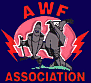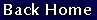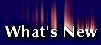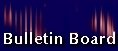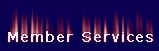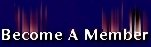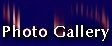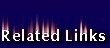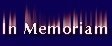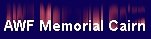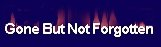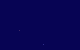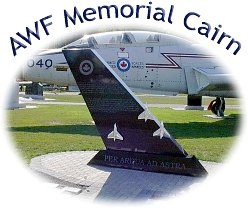"The AWF Memorial Cairn was dedicated at the RCAF Memorial Museum, 8 Wing, Trenton on 14 September 2002 at 1400 hours. The following is a list of honorees whose names are inscribed on the AWF Memorial Cairn: Capt   R. R. Abbott  416 Squadron  F/O   S. J. Allen  423 Squadron F/O   M. C. Anderson 410 Squadron Cpl   P. J. Ardley  RCAF Station North Bay  F/O   J. M. Arsenault 423 Squadron F/O   R. A. Ashmore 445 Squadron F/O   J. R. Baer  3 AW(F) OTU Capt   L. E. Bastie  409 Squadron F/O   D. G. Bate  433 Squadron F/O   R. C. Bedard  3 AW(F) OTU F/O   R. J. Bentley  423 Squadron F/O    J.L.P.W. Berrigan CEPE F/O   E. N. Bilton  414 Squadron F/L   R. W. Bogle  ADCHQ S/L   L. A. Bolin  432 Squadron Capt   R. J. Borland  425 Squadron F/L    H. H. Bouius  425 Squadron F/O   J.A.F.G  Boutin 433 Squadron Capt   P. M. Bow  410 Squadron F/O    L. J. E.  Buckman 433 Squadron Capt    T. M. Campbell 414 Squadron F/O   D. G. Carter  423 Squadron F/O   E. A. Charles  3 AW(F) OTU F/O   D. R. Clark  416 Squadron F/O   G. A. Cooling  423 Squadron Capt    L. J. Cox  409 Squadron F/O   S. W. Cratchley 409 Squadron F/L   J. R. Curtis  CEPE F/O   F. M. Dakin  3 AW(F) OTU F/O   J.G.G.G. Dallaire 414 Squadron F/O   G. M. Davis  409 Squadron F/O   J. M. Dawson  414 Squadron Lt (USAF)  R. A. De Genova 438th FIS F/O   J.R.R.G. . Desrochers 3 AW(F) OTU F/O   G. A. Donald  419 Squadron F/O   R. B. Donald  440 Squadron F/O    R. C. Dougall  432 Squadron Capt   J. A. Emon  409 Squadron F/L   K. M. Eyolfson 445 Squadron F/L   J.S.N. Findlay  3 AW(F) OTU F/L    H.A.K. Fisher 3 AW(F) OTU F/O   P. A. Flannery  433 Squadron F/O   R. R. Fletcher  414 Squadron F/O   S. S. Franko  445 Squadron F/O   D. J. Freckleton 3 AW(F) OTU F/O   R. H. Frost-Hunt 3 AW(F) OTU F/O   D.J.L. Graham 440 Squadron F/O   F.A.S. Grant  445 Squadron F/L   R. M. Grant  416 Squadron F/O   J. A. Hardy  CEPE W/C   C.E.L Hare  414 Squadron F/O   K. G. Heather  419 Squadron F/L   W. C. Henderson EWU F/O   S. A. Henry  410 Squadron Capt (USAF)  D. I. Hickman  410 Squadron Mr.    J.  Hieber  Avro Canada F/O   D. S.  Hirst  440 Squadron F/O   R. F. Holland  3 AW(F) OTU F/O   J.O.J.Y. Houde 419 Squadron Capt   G. J. Hunt  414 Squadron F/L   J. G.  Keith  EWU F/L   E. Keller  ADCHQ F/O   J. H. Kerr  129 A&FF F/O   B. Kirkham  423 Squadron F/O   R. W. Komar  423 Squadron Captain   J. D. Lauder  410 Squadron F/O   A. N. Leaf  433 Squadron F/O   B.G.P. Leon  428 Squadron P/O   B. I. Luck  3 AW(F) OTU Mr.   G. J. Lynes  Avro Canada F/O   A. K. Mackenzie 432 Squadron F/O   B. D. Mackenzie EWU S/L   A. MacMillan  3 AW(F) OTU F/L   S. A. Marshall 428 Squadron F/O   J. E. McCarthy 428 Squadron F/O   M. L. McLean 428 Squadron F/O   E. R. McCoy  419 Squadron F/O   J. McLaren  409 Squadron W/C   W.A.G. McLeish 440 Squadron F/L   K. P. McNulty 419 Squadron P/O   A. Miles  423 Squadron F/O   J. E. A. Miller  409 Squadron F/L   H. W. Mitchell 3 AW(F) OTU P/O   C. E. Ness  3 AW(F) OTU F/O   W. D. Ness  440 Squadron F/O   J. Nestoruk  425 Squadron F/O   L. B. Neumeyer 3 AW(F) OTU W/C   G. E. Nickerson 445 Squadron W/C   H. R. Norris  432 Squadron F/O   L. H. Ollenberger 423 Squadron Capt   A.  Oostenburg 416 Squadron F/O   G. R. Ormiston 3 AW(F) OTU Mr.   R. G. Ostrander  Avro Canada F/L   R. J. Palmer  EWU F/O   K. S. Partington 445 Squadron F/O    R. D. Perrie  3 AW(F) OTU F/O   J.A.S. Plante  419 Squadron F/O   K. G. Presnell  419 Squadron F/O   G.R.B. Rayment 423 Squadron F/O   J. S. Read  428 Squadron Mr.   J. Rivett  314 TSU P/O   J.Y.J.M.. Roberge 3 AW(F) OTU F/L   H. J. Robertson CEPE Capt   R.E.J. Robichaud 425 Squadron F/O   W. J. Schmidt  445 Squadron F/O   B. Shaw  409 Squadron F/O   D. A. Sheffield 433 Squadron F/O   A. R. Schellongovsky 423 Squadron F/L   W. H. Siegel  ADCHQ Capt   R. J. Smith  409 Squadron F/O   L.E.V. Sparrow 433 Squadron F/O   L. A. Strasser  433 Squadron F/O   G. S. Stubbs  409 Squadron F/O   H. Thiessen  445 Squadron F/O   J. Y. Theriault 425 Squadron F/O   K. D. Thomas  445 Squadron F/O   L. L. Tidball  129 A&FF P/O   J.M.Y. Turpin  3 AW(F) OTU F/O   J. Walton  440 Squadron F/L    B. Warren  Avro Canada F/O   J. W. Wilding  419 Squadron F/L   S. K. Woolley  ADCHQ F/O   A.D. Wright  423 Squadron F/O   C. R.. Wright  425 Squadron F/O   M. G. Wright  425 Squadron S/L   G. J. Zaleschuk 423 Squadron F/O   M. J. Zimmer  423 Squadron